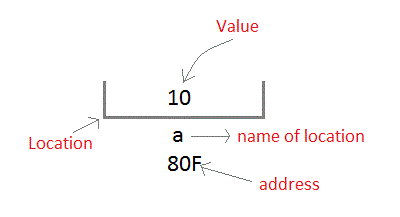# C++ Variables

In this section we will be studying the concepts of variables in C++ programming language. We hope you guys must have heard about variables in C or somewhere else. Though it is not a very complex topic to discuss in detail but let’s see what we have to say for this.

## C++ Variables

We can see a variable as a ‘source of storage with some name’, which our programs can use for modification or manipulation. There is always a type associated with each variable which we need to specify at the starting of the program during variable declaration or we can also specify the type of the variable during initialization of that particular variable.

The type of a variable determines the size of the variable’s memory ( i.e. how many bits can be accommodated within), layout of the memory, range of values that can be stored and the set of instructions that are permissible to be applied over the variable.

Things to keep in mind when naming a variable:

Digits, letters and an underscore character is what allowed in the nomenclature of the variables whereas the variables name must start with a letter or an underscore only. C++ is a case-sensitive language so the uppercase and lowercase letters are not the same.

Now let’s see what are the some basic types of variable which we can take into consideration in C++:

 Type Description int tells machine that variable is integer type char character type variable. Takes one byte or called single octet bool Boolean type which takes two values only, either ‘true’ or ‘false’ float floating type variable (takes single-precision floating value) wchar_t This stands for ‘wide character’ type double double type variable (takes double-precision floating value) void signifies the absence of any variable type

Moving on now we will study how to define, declare and use different types of variables in C++ language. Come let’s explore.

### How to Define a Variable in C++

While defining a variable in any programming language we tell the compiler where and how much space should be created for the variable. In variable definition the type of the variable and a list of one or more variables must be specified something like this:

Here type is a C++ data type that can be anything from int, char, bool, double etc. or even any user-defined data type. And the variable_list signifies one or more comma separated variable names. Let’s understand this with a code excerpt:

In the first line we declared and defined the variables a, c & num, which instructs the compiler to create three variables each of type int.

### How to Initialize a Variable in C++

Initialization basically means ‘assignment of the value’. The variable initialization can be done at the time of declaration of the variable or somewhere else in the program. Something like this:

As we can infer from above that an assignment operator ( = ) is used for the initialization of the variable. Let’s have some examples to understand this better:

We can visualize the variable a in memory as given below.Note: Keep in mind that you can declare your variable in the program as many times as you want and anywhere, but the variables can be defined only once in the file, a function or a code excerpt.

### How to Declare a Variable in C++

The declaration of the variable is for the purpose of letting compiler know that there exist a variable with given type and given name so that compiler can do it’s work i.e. further compilation without worrying about more detail of that variable.

At the time of linking of the program, the compiler do not need the variable declaration, the variable declaration does the work for it. We can infer from here that variable declaration is needed at the time of compilation only. We have seen how to declare a variable above, now let’s see an example of this:

Output

12

Above program does multiplication of two numbers.

### Local Variables

The variables that are declared inside a function or a block of code are considered as local variables. Theses variables are only confined to be used by the statements that lie within their own function or code block. The statement or function lying outside the block are not allowed to have the access to the local variable defined in other block.

In above examples of multiplication of two numbers, a, b and c where local variables because they were declared inside main() function.

### Global Variables

The variable that is defined outside all blocks and function and can be accessed by all of them is known as global variable. Global variable is usually defined at the top of the program and is available to all of the functions and blocks as long as program is into existence. And the value of the global variable is retained throughout the life of the program. Let’s have an example of code to understand this concept practically:

Output

15

Note: If there exist any such condition that both the global and the local variable have same name, then in such cases local variable is given preference over global variable.

Comment below if you have queries related to above C++ variables tutorial.

### 1 thought on “C++ Variables”

1.i am not able to understand the role of namespace std